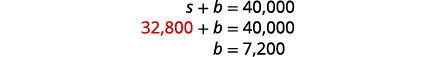# 4.4：使用方程组求解混合应用程序

•• OpenStax
• OpenStax
$$\newcommand{\vecs}{\overset { \rightharpoonup} {\mathbf{#1}} }$$ $$\newcommand{\vecd}{\overset{-\!-\!\rightharpoonup}{\vphantom{a}\smash {#1}}}$$$$\newcommand{\id}{\mathrm{id}}$$ $$\newcommand{\Span}{\mathrm{span}}$$ $$\newcommand{\kernel}{\mathrm{null}\,}$$ $$\newcommand{\range}{\mathrm{range}\,}$$ $$\newcommand{\RealPart}{\mathrm{Re}}$$ $$\newcommand{\ImaginaryPart}{\mathrm{Im}}$$ $$\newcommand{\Argument}{\mathrm{Arg}}$$ $$\newcommand{\norm}{\| #1 \|}$$ $$\newcommand{\inner}{\langle #1, #2 \rangle}$$ $$\newcommand{\Span}{\mathrm{span}}$$ $$\newcommand{\id}{\mathrm{id}}$$ $$\newcommand{\Span}{\mathrm{span}}$$ $$\newcommand{\kernel}{\mathrm{null}\,}$$ $$\newcommand{\range}{\mathrm{range}\,}$$ $$\newcommand{\RealPart}{\mathrm{Re}}$$ $$\newcommand{\ImaginaryPart}{\mathrm{Im}}$$ $$\newcommand{\Argument}{\mathrm{Arg}}$$ $$\newcommand{\norm}{\| #1 \|}$$ $$\newcommand{\inner}{\langle #1, #2 \rangle}$$ $$\newcommand{\Span}{\mathrm{span}}$$$$\newcommand{\AA}{\unicode[.8,0]{x212B}}$$

##### 学习目标

• 求解混合应用
• 解决利息申请
• 解决成本和收入函数的应用

1. 乘以：$$4.025(1,562)$$
如果你错过了这个问题，请查看 [链接]
2. 将 8.2% 写成十进制。
如果你错过了这个问题，请查看 [链接]
3. 伯爵的晚餐账单为32.50美元，他想留下18％的小费。 小费应该是多少？
如果你错过了这个问题，请查看 [链接]

## 求解混料应用程序##### 示例$$\PageIndex{1}$$

 第 1 步。 阅读问题。 我们将创建一个表来组织信息。 第 2 步。 确定我们在寻找什么。 我们正在寻找成人票的数量 和售出的儿童票数量。 第 3 步。 说出我们要找的东西。 让$$a= \text{the number of adult tickets.}$$ $$c= \text{the number of child tickets}$$ 表格将帮助我们整理数据。 我们有两种类型的门票，成人和儿童。 在 a 和 c 中写入门票数量。 在 “号码” 列的底部 写下售出的门票总数。 总共售出了 1,363 个。 在 “值” 列中写下每种类型票证的 值。 每张成人票的价值为12美元。 每张儿童票的价值为7美元。 乘以该值得出总价值， 因此成人票的总价值为$$a·12=12a$$ ，儿童票的总价值为$$c·7=7c$$。 填写总值列。 门票的总价值为12,146美元。第 4 步。 转化为方程组。 数字列和总值列 为我们提供了方程组。我们将使用消除方法来解决 这个系统。 将第一个方程乘以$$−7$$。简化并添加，然后求解 a。代$$a=521$$入第一个方程，然后 求解 c。第 6 步。 检查 问题中的答案。 521 名成人，每张票 12 美元，每张票 7 美元，儿童 6 ,252 美元 842 美元，总收入为 12,146 美元$$\checkmark$$ 第 7 步。 回答问题。 科学中心售出了 521 张成人门票和 842 张儿童票。

206 名成人，347 名儿童

42 位成人，105 位儿童

##### 示例$$\PageIndex{4}$$

 第 1 步。 阅读问题。 我们将创建一个表来组织信息。 第 2 步。 确定我们在寻找什么。 我们正在寻找 镍的数量和硬币的数量。 第 3 步。 说出我们要找的东西。 让$$n= \text{the number of nickels.}$$ $$d= \text{the number of dimes}$$ 表格将帮助我们整理数据。 我们有两种类型的硬币，镍和硬币。 写上 n 和 d 作为 每种硬币的编号。 在 “价值” 列中填写每 种硬币的价值。 每种镍的价值为0.05美元。 每角钱的价值为0.10美元。 该值的次数给出了总 价值，因此，镍的总价值为 $$n(0.05)=0.05n$$，毛钱的总价值为 $$d(0.10)=0.10d$$。 这些硬币的总价值为8.10美元。第 4 步。 转化为方程组。 总值列给出一个等式。我们还知道硬币的数量是9分比镍的 两倍少。 翻译得到第二个方程。现在我们有系统要解决了。第 5 步。 求解方程组 我们将使用替换方法。 代$$d=2n−9$$入第一个方程式。简化并求解 n。要找到毛钱的数目，请用第二个 $$n=36$$方程代替。第 6 步。 在 Total 查看问题中的答案 63 毛钱$$0.10=6.30$$ 36$$0.05=1.80$$ 个镍$$=8.10\checkmark$$ 第 7 步。 回答问题。 胡安有 36 个镍和 63 角钱。

13 毛钱和 29 个季度

##### 示例$$\PageIndex{6}$$

Priam拥有一系列镍和四分之一，总价值为7.30美元。 镍的数量是六个，少于季度数的三倍。 他有多少镍和多少个季度？

19 个四分之一和 51 个镍

##### 示例$$\PageIndex{7}$$

 第 1 步。 阅读问题。 我们将创建一个表来组织信息。 第 2 步。 确定我们在寻找什么。 我们正在寻找 坚果的磅数和巧克力 片的磅数。 第 3 步。 说出我们要找的东西。 让$$n= \text{the number of pound of nuts.}$$ $$c= \text{the number of pounds of chips}$$ Carson 会混合坚果和巧克力片来 混合。 用 n 和 c 写下 坚果和巧克力片的磅数。会有 20 磅的越野混合物。 在 “价值” 列中输入每件商品 的每磅价格。 使用填写最后一列 $$\text{Number}•\text{Value}=\text{Total Value}$$ 第 4 步。 转化为方程组。 我们从 “数字” 和 “总值” 列中获得方程。第 5 步。 求解方程组 我们将使用消除来求解方程组。 将第一个方程乘$$−2$$以消除 c。简化并添加。 求解 n。要找到巧克力 片的磅数，请代$$n=16$$入第一个方程， 然后求解 c。第 6 步。 检查问题中的答案。 $$\begin{array} {lll} 16+4 &= &20\checkmark \\ 9·16+2·4 &= &152\checkmark \end{array}$$ 第 7 步。 回答问题。 Carson 应该将 16 磅的坚果和 4 磅的巧克力片混合，制成 混合物。
##### 示例$$\PageIndex{8}$$

Greta 想用花生和腰果制成 5 磅的坚果混合物。 她的预算要求混合物每磅花费6美元。 花生为每磅4美元，腰果为每磅9美元。 她应该用多少磅花生和多少磅腰果？

3 磅花生和 2 磅腰果

10 磅豆子，10 磅碎牛肉

##### 示例$$\PageIndex{10}$$

 第 1 步。 阅读问题。 一个数字可以帮助我们可视化 情况，然后我们将创建一个表格来 组织信息。 Sasheena 必须将 一些$$25%$$解决方案和一些$$50%$$解决方案混合在一起才能得到$$200\space ml$$$$40%$$解决方案。第 2 步。 确定我们在寻找什么。 我们正在寻找她 需要多少解决方案。 第 3 步。 说出我们要找的东西。 让$$x= \text{number of }ml\text{ of }25% \text{ solution.}$$ $$y= \text{number of }ml\text{ of }50%\text{ solution$$ 表格将帮助我们整理数据。 她会将 x o$$ml$$ f 和 y o$$ml$$ f 混在$$25%$$一起$$50%$$$$200 \space ml$$ 以获得$$40%$$解决方案。 我们在图表中将百分比写 成小数。 我们将单位数乘以 浓度得出每种溶液中的 硫酸总量。第 4 步。 转换为 方程组。 我们从 “数字 ” 列和 “金额” 列中获得方程。 现在我们有了系统。第 5 步。 求解方程组 我们将通过消除来求解方程组。 将第一个方程乘$$−0.5$$以 消除 y。简化并添加以求解 x。要求解 y，请$$x=80$$将其替换为第一个 方程。第 6 步。 检查问题中的答案。 $$\begin{array} {lll} 80+120 &= &200\checkmark \\ 0.25(80)+0.50(120) &= &200\checkmark \\ {} &{} &\text{Yes!} \end{array}$$ 第 7 步。 回答问题。 Sasheena 应该将$$25%$$解决方案与 $$120 \space ml$$$$50%$$溶液混合在一起$$80 \space ml$$，$$200\space ml$$以获得 $$40%$$解决方案。
##### 示例$$\PageIndex{11}$$

120 毫升 25% 溶液和 30 毫升 50% 溶液

##### 示例$$\PageIndex{12}$$

125 毫升 10% 溶液和 125 毫升 40% 溶液

## 解决利息申请

 第 1 步。 阅读问题。 图表将帮助我们整理信息。 第 2 步。 确定我们在寻找什么。 我们正在寻找每只基金的投资金额。 第 3 步。 说出我们要找的东西。 让$$s= \text{the amount invested in stocks.}$$ $$b= \text{the amount invested in stocks}$$ 将 每只基金的利率写成小数。 乘以：本金·费率·时间第 4 步。 转换为 方程组。 我们从 “本金” 列和 “ 利息” 列中获得方程组。第 5 步。 通过消法求解方程组。 将顶部方程乘以$$−0.03$$。简化并添加以求解 s。要找出 b，请将 s = 32,800 代 入第一个方程。第 6 步。 检查 问题中的答案。 我们把支票留给你。 第 7 步。 回答问题。 阿德南应该投资32,800 美元的股票和7,200美元的债券。

##### 示例$$\PageIndex{15}$$

1750 美元，11％，5250 美元，13％

##### 示例$$\PageIndex{16}$$

 第 1 步。 阅读问题。 图表将帮助我们整理信息。 第 2 步。 确定我们在寻找什么。 我们正在寻找每笔贷款的本金。 第 3 步。 说出我们要找的东西。 让$$b= \text{the principal for the bank loan.}$$ $$f= \text{the principal on the federal loan}$$ 贷款总额为21,540美元。 在图表 中将利率记录为小数。 使用公式 I = P r t 进行乘以 得利息。第 4 步。 转换为 方程组。 方程组来自 “ 本金” 列和 “利息 ” 列。第 5 步。 求解方程组 我们将使用替换来求解。 求解 b 的第一个方程。将 b = − f + 21.540 代入 第二个方程。简化并求解 f。要找出 b，请将 f = 12,870 替换到第一个方程中。第 6 步。 检查 问题中的答案。 我们把支票留给你。 第 7 步。 回答问题。 联邦贷款的本金为12,870美元， 银行贷款的本金为8,670美元。

##### 示例$$\PageIndex{18}$$

Jill's Sandwich Shoppe欠两笔商业贷款65,200美元，一笔利息为4.5％，另一笔利息为7.2％。 去年所欠利息总额为3582美元。 每笔贷款的本金是多少？

41,200 美元，4.5％，24,000 美元，7.2％

## 解决成本和收入函数的应用

##### 成本和收入函数

$C(x)=(\text{cost per unit})·x+\text{fixed costs}\nonumber$

$R(x)=(\text{selling price per unit})·x\nonumber$

$C(x)=R(x)\nonumber$

##### 示例$$\PageIndex{19}$$

ⓐ 在制造 x 长凳时找到成本函数 C

ⓑ 当售出 x 个长凳时，找到收益函数 R

ⓒ 通过在同一个网格上绘制收入和成本函数的图表来显示盈亏平衡点。

ⓓ 找到盈亏平衡点。 解释盈亏平衡点的含义。

ⓐ 无论生产多少举重训练台，制造商都有7,000美元的固定成本。 除了固定成本外，制造商还花费105美元生产每个工作台。 假设已售出 x 个长凳。

$$\begin{array} {ll} {\text{Write the general Cost function formula.}} &{C(x)=(\text{cost per unit})·x+\text{fixed costs}} \\ {\text{Substitute in the cost values.}} &{C(x)=105x+7000} \\ \end{array}$$

ⓑ 制造商以245美元的价格出售每个举重训练台。 我们通过将每单位收入乘以售出单位数得出总收入。

$$\begin{array} {ll} {\text{Write the general Revenue function.}} &{C(x)=(\text{selling price per unit})·x} \\ {\text{Substitute in the revenue per unit.}} &{R(x)=245x} \\ \end{array}$$

ⓒ 本质上我们有一个线性方程组。 我们将展示系统的图表，因为这有助于使盈亏平衡点的概念更加直观。

$\left\{ \begin{array} {l} C(x)=105x+7000 \\ R(x)=245x \end{array} \right. \quad \text{or} \quad \left\{ \begin{array} {l} y=105x+7000 \\ y=245x \end{array} \right. \nonumber$ⓓ 为了找到实际价值，我们记得当成本等于收入时出现盈亏平衡点。

$$\begin{array} {ll} {\text{Write the break-even formula.}} &{\begin{array} {l} {C(x)=R(x)} \\ {105x+7000=245x} \end{array}} \\ {\text{Solve.}} &{\begin{array} {l} {7000=140x} \\ {50=x} \end{array}} \\ \end{array}$$##### 示例$$\PageIndex{20}$$

ⓐ 在制造 x 长凳时找到成本函数 C

ⓑ 当售出 x 个长凳时，找到收益函数 R

ⓒ 通过在同一个网格上绘制收入和成本函数的图表来显示盈亏平衡点。

ⓓ 找到盈亏平衡点。 解释盈亏平衡点的含义。

$$C(x)=15x+25,500$$

$$R(x)=32x$$ⓓ 1,5001,500；当售出 1,500 个长凳时，成本和收入将均为 48,000

##### 示例$$\PageIndex{21}$$

ⓐ 在制造 x 长凳时找到成本函数 C

ⓑ 当售出 x 个长凳时，找到收益函数 R

ⓒ 通过在同一个网格上绘制收入和成本函数的图表来显示盈亏平衡点。

ⓓ 找到盈亏平衡点。 解释盈亏平衡点的含义。

$$C(x)=120x+150,000$$

$$R(x)=170x$$$$3,000$$; 当售出 3,000 个长凳时，收入和成本均为 510,000 美元

• 利息和混合物

## 关键概念

• 成本函数：成本函数是每个单位的制造成本乘以 x、制造的单位数加上固定成本。

$$C(x)=(\text{cost per unit})·x+\text{fixed costs}$$

• 收入：收入函数是每个单位的销售价格乘以 x，即售出的单位数。

$$R(x)=(\text{selling price per unit})·x$$

• 盈亏平衡点：盈亏平衡点是收入等于成本的时候。

$$C(x)=R(x)$$